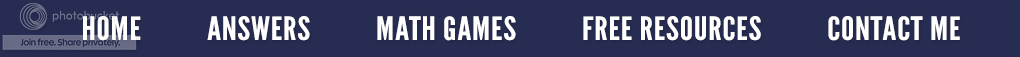### Slope for Vertical and Horizontal LinesI work in the Math Lab at the community college where I also teach. Last week, I had two College Algebra students who were having difficulty with slope.  They knew the equation y = mx + b, but were unsure when it came to horizontal or vertical lines. By the way, they were using their graphing calculators which I made them put away. (The book said no calculators.) I feel that if they construct the lines themselves, it puts a visual image into their brain much better than if the calculator does it for them. Sure enough, one of the sections in their math books gave the picture of the line from which they had to write the equation. They were amazed that I could just look at a graph and know the slope, give the equation, etc. When I taught high school math, my students couldn't use a graphing calculator until the middle of this particular chapter as I wanted them to physically draw the lines.

First, for those who have no idea what I am talking about, slope is rise over run.  Rise is how far a line goes up, and run is how far a line goes along.  At the right, the line goes up 3 and has a run 5; therefore, the slope is 3/5.  Rise/Run (Rise divided by Run) gives us the slope of the line.When a line is horizontal, it has no rise, only a run. So the numerator would be zero (for no rise) and the denominator would be a number such as 5 for the run.  0 ÷ 5 = 0  This is true for any horizontal line.A vertical line is different.  It has rise, but no run; therefore there would always be a number in the numerator, but always a zero in the denominator.  Since we cannot divide by zero, the slope is considered undefined. (I do use rise over run stating that a horizontal line might have 0/5 which is equal to 0 and that a vertical line might have 3/0 is undefined because we can't divide by zero. Our college algebra book uses O/K for okay and K/O for knock out which I like, but I still think the students need to know why.)

I wanted these two students to have a picture that would help them remember the difference.  I thought of a table for the horizontal line and asked them what would happen if the legs of the table were uneven.  They agreed that the table would have slope.  Therefore, the table would have a slope of zero if the legs were even.

I then went blank.  In other words, by creative juices stopped working, and I could not think of a picture that would help them visualize undefined. Since Teachers Pay Teachers has a forum,, I asked my fellow math teachers if they had any ideas.  Here is what some of them came up with.

The Enlightened Elephant suggested using a ski slope. She talks about skiing down a "cliff", which would not be possible (although some students try to argue that they could ski down a vertical cliff) and so the slope is "undefined" because it doesn't make sense to ski down a cliff.  Skiing on a horizontal line is possible so it's slope is zero,  She also talks about uphill (positive slope) and downhill (negative slope).

Math by Lesley Elisabeth tells her students to use "HOY VUX" (rhymes with 'toy bucks')

Horizontal - Zero (0) slope - y = ?
Vertical - Undefined slope - x = ?

All horizontal lines are =7 or = -3 etc., and all vertical lines are =1 or = 6, etc. Students forget this so the acronym HOY VUX helps them to remember. Once they've mastered the slope concept in Algebra I, for the rest of the school year, for Algebra II (especially equations of asymptotes - a line that continually approaches a given curve but does not meet it at any finite distance) and even in calculus classes for tangent lines, HOY VUX is just faster and more practical.

created a video lesson on the Slope Intercept  (\$5 on TPT).  She has a boy skateboard down a negative slope, literally right on the graph line. Karen then shows the same boy taking an escalator up on a line that has a positive slope. Later in the lesson, she rotates the line clockwise, each movement with a click, to show the corresponding slope number to link the line to the slope.  She includes lots of other visual cues to help students focus on and pay attention to the concepts.

I did find a video on Pinterest that might help us all. It's called Slope Dude.  My students thought it was corny, but it did help them to remember.

Take some time to check some other great blog posts by my
fellow members of The Best of Teacher Entrepreneurs.